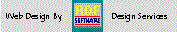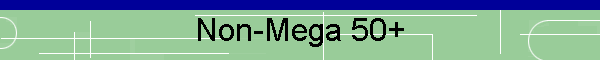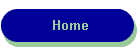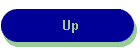RDF-Home LUGIE Linux Users Group Zenbot CNC User's Group The first Super Lotto Plus game {#1375} was drawn on June 7th. 2000.  To play this NEW game, you still pick 6 numbers, but choose 5 numbers in the range of 1 to 47 and then pick a number in the range of 1 to 27 as the mega Number.  This analysis looks only at the Non-Mega Number frequency part of the game. The chart below shows the frequency of numbers selected from the most recent Pick-6 Super Lottery Drawings: Game #3104  through 3050  excluding mega-numbers,  Updated 01/05/17      Check out Mega Number frequencies here!         Non-Mega Number Frequency for the California State Lottery           Num=  1 Count=   7    Num= 25 Count=   7           Num=  2 Count=   6    Num= 26 Count=   3           Num=  3 Count=   8    Num= 27 Count=   5           Num=  4 Count=   4    Num= 28 Count=   6           Num=  5 Count=   4    Num= 29 Count=   6           Num=  6 Count=   6    Num= 30 Count=   8           Num=  7 Count=   5    Num= 31 Count=   7           Num=  8 Count=   6    Num= 32 Count=   5           Num=  9 Count=   4    Num= 33 Count=   4           Num= 10 Count=   8    Num= 34 Count=   7           Num= 11 Count=   5    Num= 35 Count=   5           Num= 12 Count=   5    Num= 36 Count=   6           Num= 13 Count=   7    Num= 37 Count=   9           Num= 14 Count=   3    Num= 38 Count=   3           Num= 15 Count=   8    Num= 39 Count=  10           Num= 16 Count=   6    Num= 40 Count=   7           Num= 17 Count=   4    Num= 41 Count=   6           Num= 18 Count=   6    Num= 42 Count=   4           Num= 19 Count=   2    Num= 43 Count=   6           Num= 20 Count=   7    Num= 44 Count=   8           Num= 21 Count=   7    Num= 45 Count=   5           Num= 22 Count=   4    Num= 46 Count=   8           Num= 23 Count=   6    Num= 47 Count=   7           Num= 24 Count=   5 Valid Numbers in this series=275 from 55 most recent Games Frequency Range:  Max=10 Min=2      Most Frequent Numbers:           Number   39 Frequency=  10      Target Frequency:   7           Number    1 Frequency=   7           Number   13 Frequency=   7           Number   20 Frequency=   7           Number   21 Frequency=   7           Number   25 Frequency=   7           Number   31 Frequency=   7           Number   34 Frequency=   7           Number   40 Frequency=   7           Number   47 Frequency=   7      Least Frequent Numbers:           Number   14 Frequency=   3           Number   26 Frequency=   3           Number   38 Frequency=   3           Number   19 Frequency=   2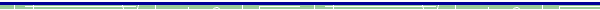dBase, Delphi  and C++ Builder  are trademarks of Borland International, Inc. All other products mentioned are registered trademarks or trademarks of their respective companies. Questions or problems regarding this web site should be directed to RDF@RDFoerster.com. Copyright © 1997-2007 RDFoerster Software. All rights reserved. Last modified: Thursday January 05, 2017